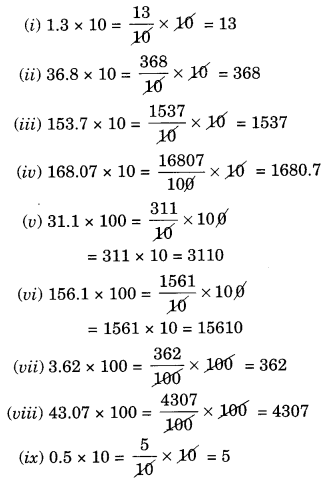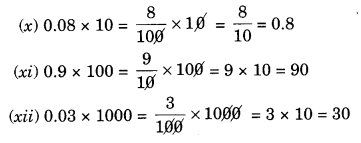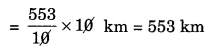• +91 9971497814
• info@interviewmaterial.com

# Chapter 2 Fractions and Decimals Ex-2.6 Interview Questions Answers

### Related Subjects

Question 1 :
Find:
(i) 0.2 × 6
(ii) 8 × 4.6
(iii) 2.71 × 5
(iv) 20.1 × 4
(v) 0.05 × 7
(vi) 211.02 × 4
(vii) 2 × 0.86

(i) 0.2 × 6
∵ 2 × 6 = 12 and we have 1 digit right to the decimal point in 0.2.
Thus 0.2 × 6 = 1.2
(ii) 8 × 4.6
∵ 8 × 46 = 368 and there is one digit right to the decimal point in 4.6.
Thus 8 × 4.6 = 36.8
(iii) 2.71 × 5
∵ 271 × 5 = 1355 and there are two digits right to the decimal point in 2.71.
Thus 2.71 × 5 = 13.55
(iv) 20.1 × 4
∵ 201 × 4 = 804 and there is one digit right to the decimal point in 20.1.
∵ 20.1 × 4 = 80.4
(v) 0.05 × 7
∵ 5 × 7 = 35 and there are 2 digits right to the decimal point in 0.05.
Thus 0.05 × 7 = 0.35
(vi) 211.02 × 4
∵ 21102 × 4 = 84408 and there are 2 digits right to the decimal point in 211.02.
Thus 211.02 × 4 = 844.08
(vii) 2 × 0.86
∵ 2 × 86 = 172 and there are 2 digits right to the decimal point in 0.86.
Thus 2 × 0.86 = 1.72

Question 2 : Find the area of rectangle whose length is 5.7 cm and breadth is 3 cm.

Length = 5.7 cm
Area of rectangle = length × breadth
= 5.7 × 3 = 17.1 cm2
Hence, the required area =17.1 cm2

Question 3 :
Find:
(i) 1.3 × 10
(ii) 36.8 × 10
(iii) 153.7 × 10
(iv) 168.07 × 10
(v) 31.1 × 100
(vi) 156.1 × 100
(vii) 3.62 × 100
(viii) 43.07 × 100
(ix) 0.5 × 10
(x) 0.08 × 10
(xi) 0.9 × 100
(xii) 0.03 × 1000Question 4 : A two-wheeler covers a distance of 55.3 km in one litre of petrol. How much distance will it cover is 10 litres of petrol?

Distance covered in 1 litre = 55.3 km
Distance covered in 10 litres = 55.3 × 10 kmHence, the required distance = 553 km

Question 5 :
Find:
(i) 2.5 ×0.3
(ii) 0.1 × 51.7
(iii) 0.2 × 316.8
(iv) 1.3 × 3.1
(v) 0.5 × 0.05
(vi) 11.2 × 0.15
(vii) 1.07 × 0.02
(viii) 10.05 × 1.05
(ix) 100.01 × 1.1
(x) 100.01 × 1.1

(i) 0 2.5 × 0.3
∵ 25 × 3 = 75 and there are 2 digits (1 + 1) right to the decimal points in 2.5 and 0.3.
Thus 2.5 × 0.3 = 0.75
(ii) 0.1 × 51.7
∵ 1 × 517 = 517 and there are two digits (1 + 1) right to the decimal places in 0.1 and 51.7.
(iii) 0.2 × 316.8
∵ 2 × 3168 = 6336 and there are 2 digits (1 + 1) right to the decimal points in 0.2 and 316.8.
Thus 0.2 × 316.8 = 63.36.
(iv) 1.3 × 3.1
∵ 13 × 31 = 403 and there are 2 digits (1 + 1) right to the decimal points in 1.3 and 3.1.
Thus 1.3 × 3.1 – 4.03
(v) 0.5 × 0.05
∵ 5 × 5 = 25 and there are 3 digits (1 + 2) right to the decimal points in 0.5 and 0.05.
Thus 0.5 × 0.05 = 0.025
(vi) 11.2 × 0.15
∵ 112 × 15 = 1680 and there are 3 digits (1 + 2) right to the decimal points in 11.2 and 0.15.
Thus 11.2 × 0.15 = 1.680
(vii) 1.07 × 0.02
∵ 107 × 2 = 214 and there are 4-digits (2 + 2) right to the decimal places is 1.07 × 0.02.
Thus 1.07 × 0.02 = 0.0214
(viii) 10.05 × 1.05
∵ 1005 × 105 = 105525 and there are 4 digits (2 + 2) right to the decimal places in 10.05 × 1.05.
Thus 10.05 × 1.05 = 10.5525
(ix) 101.01 × 0.01
∵ 10101 × 1 = 10101 and there are 4 digits (2 + 2) right to the decimal places in 101.01 and 0.01.
Thus 101.01 × 0.01 = 1.0101
(x) 100.01 × 1.1
∵ 10001 × 11 = 110011 and there are 3 digits (2 + 1) right to the decimal points in 100.01 and 1.1.
Thus 100.01 × 1.1 = 110.011.

Todays Deals### Chapter 2 Fractions and Decimals Ex-2.6 Contributorskrishan

Name:
Email:

# Latest News# 9000 interview questions in different categories